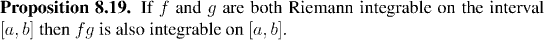Analysis WebNotes
arrow_back arrow_forward

# Class Contents

## More Properties of the Integral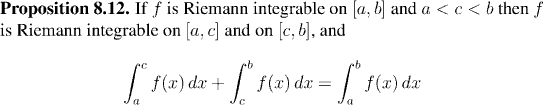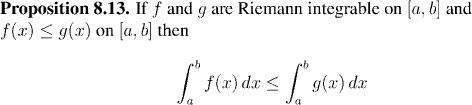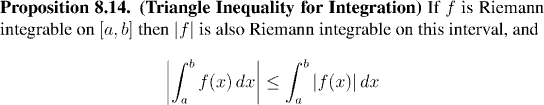## The Indefinite Integral, and Another Form of the Fundamental Theorem

In calculus, one learns how to form "the integral" of a function. Given a function, its integral is viewed as another function. It seems that our definition of integration covers only definite integrals, where the integral of a function is computed over a specified range, and a number is returned. However, it is easy to use our current understanding to define the indefinite integral as well: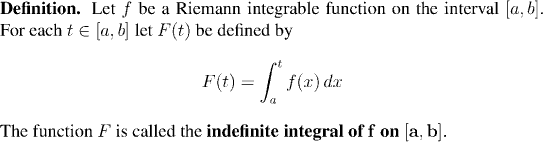Remark:
Proposition 8.12 shows that the function F(x) is well-defined for all x in [a,b].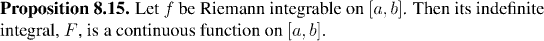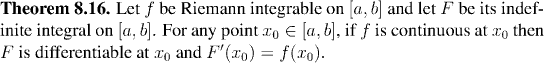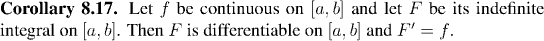## Riemann Integrable Functions

We know that all continuous functions are Riemann integrable, but we also know from the result with step functions that some non-continuous functions can be integrable. In fact quite a large family of functions is integrable, and in this section we shall investigate the properties of integrable functions.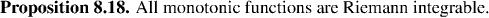We already know that sums and scalar multiples of Riemann integrable functions on the same interval are themselves Riemann integrable. This means that the set of all Riemann integrable functions on a given interval is a vector space.

In fact also, products of integrable functions are also integrable. Of course the integral of the product of two functions is not related to the integrals of the functions in a simple fashion, but it can still be useful to know that the property of integrability is preserved by multiplication.